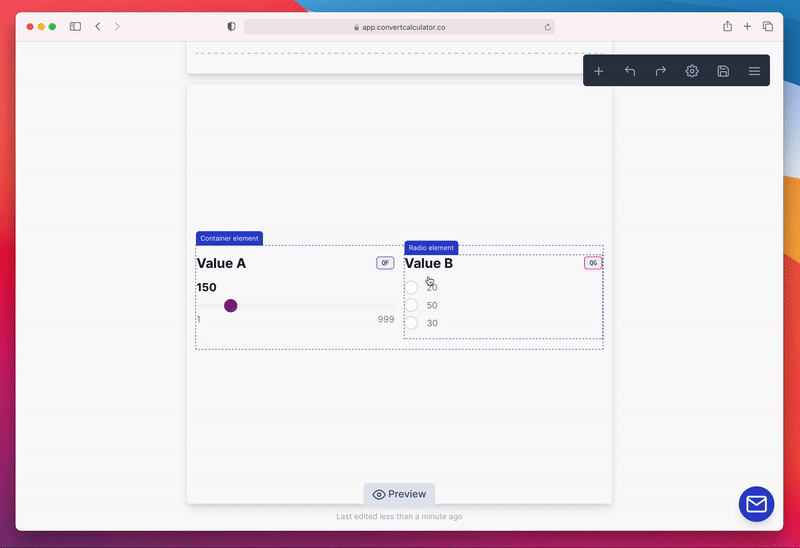# Formulas

Although not visible on the surface, ConvertCalculator offers a lot of power to add both simple and complex calculations. This article explains some of these calculations.

ConvertCalculator distinguishes three types of form elements: input elements (e.g., number question, option question, etc.), output elements, and static elements (text-field, header, button, etc). A Formula is an output element, together with tables and layered images.

The formula element is used to display a result, e.g., price or calculated value, in your calculation form.# How does a formula work?

A formula can have the following components:

• numbers: (`1, 12, 1.5, 20.5`)
• operators: (`+, -, *, /, ^`)
• question values: (`QA, QB, QC`)
• variable values: (`VA, VB, VC`)
• datasheet values: (`DSA_A, DSA_B`)

The first two need no explanation. Question values are the values users input themselves and are based on the assigned values in the calculator questions. E.g., if you ask for desired "width" or "finish", you can assign values to these questions and use these values to calculate something like price or savings.

An example of a simple formula is this:

`FS`QA * 100 + 100``

In this formula, we multiply the value of Question A by 100 and add 100. When a user changes the value of QA (for example, by sliding the range slider), the value of the formula changes automatically.

# Common issues

To successfully calculate outcomes, your formula syntax must follow certain 'rules'. The following scenarios will generate errors in your formula:

• Numbers with comma decimals (e.g `1,1` or `1,000.54`) will NOT work.
• Numbers with decimals without a leading zero (e.g `.76`) will NOT work.
• Using `=` before a formula causes the formula to throw an ERROR.
• Dividing by 0 causes the formula to throw an ERROR.

# Using Functions

Functions can make your formulas even more powerful. A function is an expression that calculates a value. A function can have input arguments, and it returns an output. The `IF function` is a perfect example of a function that can be used inside a formula:

``IF(QA > 50, QA * 2, 0)``
With ConvertCalculator you can use all common functions that you might already know from your favorite spreadsheet program. See the functions page for a full list of supported functions.

## Related articles